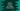# Convert an integer or float to hex in Python## Convert an integer or float to hex in Python :

In this tutorial, we will learn how to find out the hex value of an integer or a float in python.Hexadecimal is a base 16 number system. It uses the numbers 0 to 9 and then uses the letters_ A to F_ for representation. Numbers from zero to nine are represented by ‘0’ to ‘9’ like decimal._ ‘A’ to ‘F’_ are used to represent values from ten to fifteen.

To convert a decimal value to hexadecimal, we need to do a lot of calculations. But python provides us two inbuilt methods to find out the hexadecimal representation of an integer or for a float number.

In this blog post, we are not going to write the complete process for converting a number to hexadecimal. Instead, we will learn how to generate the hexadecimal value using the provided inbuilt methods.

## Using hex() method :

Method hex() is used to find out the hex value of an integer. It converts an integer number to a lowercase hexadecimal string. The hexadecimal string is prefixed with ‘0x’. One more thing to note that we can even find out the hexadecimal value of a negative integer. The syntax of this method is like below :

``hex(x)``

It takes only one parameter. The parameter should be an int number or it should define a index() method that returns an integer value. It will convert the provided integer value to hexadecimal and returns the result in string format.

Let me show you with one example of how it works :

As you can see that 0x is prefixed with all strings indicating that the string is a hexadecimal representation. Now, let’s try to find out the hex value of a float using the same method :

That means we cannot use this method for float numbers.

## Finding hex values for float :

As we have seen above, we cannot use hex() method to find the hex values for floating numbers. To find the hexadecimal value for floating numbers in python, we can use float.hex() method. This method takes one float as an input argument and returns the hexadecimal string as like below.

Example : ‘float.hex()’ method is used to convert a floating point number to its hexadecimal value. Similarly, we can use_ ‘float.fromhex()’_ method to convert a hexadecimal string value to its floating point representation._ ‘hex()’_ is an instance method but ‘fromhex()’ is a class method.

### ToDo :

We can use the above methods to create one simple hexadecimal converter in python. I am writing down the basic steps below.

1. Create one python file. We will write our converter code in it.
2. Ask the user to enter a number.
3. Check if it is a valid number or not. If not, print one error message and exit.
4. Check if the number is an integer number or floating point number.
5. If it is an integer, use ‘hex(x)’ and if it is a float, use ‘float.hex()’ to find out the hex value.
6. Print out the result to the user.

### Conclusion :

We have learned how to find the hexadecimal values of integer and floating point numbers in python. Try to run the examples explained above and drop one comment below if you have any queries.